The Australian Journal of Mathematical Analysis and Applications

 Home News Editors Volumes RGMIA Subscriptions Authors Contact

ISSN 1449-5910

You searched for yousef
Total of 26 results found in site

13: Paper Source PDF document

Paper's Title:

Para-chaotic Tuples of Operators

Author(s):

Department of Mathematics,
Payame Noor University,
P.O. Box 19395-3697, Tehran,
Iran
b_yousefi@pnu.ac.ir

Abstract:

In this paper, we introduce para-chaotic tuples of operators and we give some relations between para-chaoticity and Hypercyclicity Criterion for a tuple of operators.

5: Paper Source PDF document

Paper's Title:

On the Hyers-Ulam Stability of Homomorphisms and Lie Derivations

Author(s):

Department of Mathematics, Payame Noor University,
P.O. Box: 19395-3697, Tehran,
Iran.

Abstract:

Let A be a Lie Banach*-algebra. For each elements (a, b) and (c, d) in A2:= A * A, by definitions

(a, b) (c, d)= (ac, bd),
|(a, b)|= |a|+ |b|,
(a, b)*= (a*, b*),

A2 can be considered as a Banach*-algebra. This Banach*-algebra is called a Lie Banach*-algebra whenever it is equipped with the following definitions of Lie product: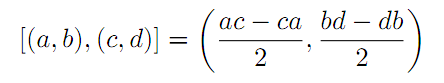for all a, b, c, d in A. Also, if A is a Lie Banach*-algebra, then D: A2→A2 satisfying

D ([ (a, b), (c, d)])= [ D (a, b), (c, d)]+ [(a, b), D (c, d)]

for all \$a, b, c, d∈A, is a Lie derivation on A2. Furthermore, if A is a Lie Banach*-algebra, then D is called a Lie* derivation on A2 whenever D is a Lie derivation with D (a, b)*= D (a*, b*) for all a, b∈A. In this paper, we investigate the Hyers-Ulam stability of Lie Banach*-algebra homomorphisms and Lie* derivations on the Banach*-algebra A2.

5: Paper Source PDF document

Paper's Title:

Some Properties on a Class of p-valent Functions Involving Generalized Differential Operator

Author(s):

A. T. Yousef, Z. Salleh and T. Al-Hawary

Department of Mathematics,
Faculty of Ocean Engineering Technology and Informatics, Universiti Malaysia Terengganu,
21030 Kuala Nerus, Terengganu,
Malaysia.
E-mail: abduljabaryousef@gmail.com, zabidin@umt.edu.my

Department of Applied Science,
Ajloun College, Al-Balqa Applied University,
Ajloun 26816,
Jordan.
E-mail: tariq_amh@yahoo.com

Abstract:

This paper aiming to introduce a new differential operator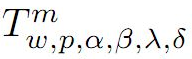in the open unit disc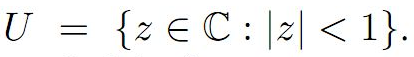We then, introduce a new subclass of analytic function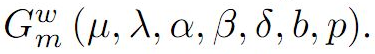Moreover, we discuss coefficient estimates, growth and distortion theorems, and inclusion properties for the functions belonging to the class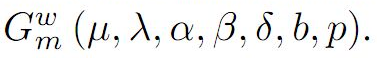3: Paper Source PDF document

Paper's Title:

Coefficient Estimates Of Sakaguchi Kind Functions Using Lucas Polynomials

Author(s):

H. Priya and B. Srutha Keerthi

Department of Mathematics,
VIT Chennai Campus,
Chennai - 600 048,
India.
E-mail: priyaharikrishnan18@gmail.com

Department of Mathematics,
VIT Chennai Campus,
Chennai - 600 048,
India.
E-mail: i
sruthilaya06@yahoo.co.in

Abstract:

By means of (p,q) Lucas polynomials, we estimate coefficient bounds and Fekete-Szego inequalities for functions belonging to this class. Several corollaries and consequences of the main results are also obtained.

Search and serve lasted 0 second(s).